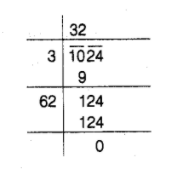# Find the number of plants in each row,

Question:

Find the number of plants in each row, if 1024 plants are arranged, so

that number of plants in a row is the same as the number of rows.

Solution:

Let the number of plants in each row be $x$.

Then, number of rows $=$ Number of plants in each row $=x$$\therefore \quad$ Total plants $=x \times x=x^{2}$

According to the question,

$x^{2}=1024$

$\Rightarrow \quad x=\sqrt{1024}=\sqrt{32 \times 32}$

$\therefore \quad x=32$

Hence, there are 32 plants in each row.